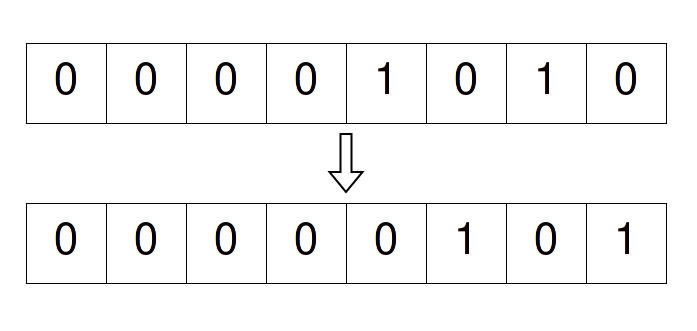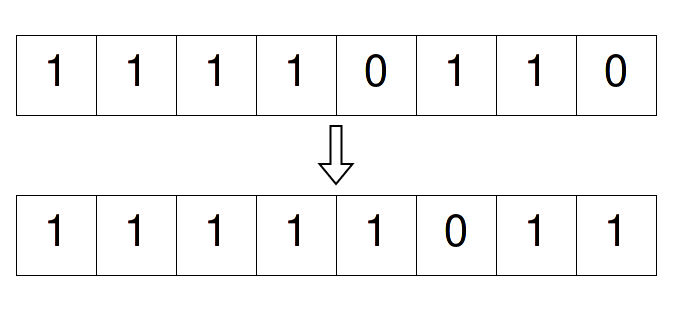×

Search anything:

# Absolute value of Integer using Bitwise Operations

#### Algorithms bitwise algorithmIn this article, we have explained two approaches to find the Absolute value of Integer using Bitwise Operations namely Right shift, OR and XOR operations.

# Introduction

If we define what's Absolute value, then it says that "The distance of some number from the zero is an Absolute Value".
Which means if there is some given number +/- N then the Absolute Value of this number should be N.

One way to find out the Absolute value of a number will be to check if the given value is `negative` or `positive` and if it's `positive`, then return `n` and if it's `negative`, then return `n - 2*n`.

Code for the above Example:

``````fn abs(n: i8) -> i8 {
if n > 0 {
n
} else {
(n - n) - n // Using this because,
// Above mentioned can overflow.
}
}
``````

But, you see, this requires an `if-else` statement, and if we used `binary operations` to find Absolute Value, then one can avoid using `if-else` statements.

Note: we will be considering only 8-bit numbers here, but you can apply this method to others as well.
Just use `size_of(n) * 8` to find out the number of bits for the data type you're using.

# Integers in binary

I think if you're scrolling through this page, then you should already be familiar with how we represent integers in binary and how we know if a number is `positive` or `negative` but, to make sure that we're on the same page, we'll look at it once again.

There are two kinds of numbers one is `signed` and `unsigned`.

### `unsigned`

• The range for `unsigned` numbers goes simply from `0 to 255` and in binary `0000_0000` to `1111_1111`.

### `signed`

• But the range for `signed` numbers goes `from -128 to 127`, and in binary, there are two ranges to make note of

• `0 to 127` -> `0000_0000` to `0111_1111`
• `-1 to -128` -> `1111_1111` to `1000_0000`

Yep!! It counts backward.

And Of course, we will be using only `signed` integers here.

# Shifting Bits

The rule is to have a 1 bit (the most left one) in signed integers as a sign bit, when it's `0` then, consider the number to be `positive` and `negative` if the sign bit is `1`.

Now, shifting bits to the left will do the same things for both `positive` and `negative` integers.

But when we right shift the bits then, a `positive` integer will have no issue and, there will be a `0` in the sign bit before and even after the shift.
But, when we right shift a `negative` integer then, it is necessary that we make sure to preserve the sign bit. So, there will be `1` present in the sign bit.

For Example:

### Positive Number

Let's consider a number `n = 10` and, now if we right shift it, then it should look something like the given diagram:### Negative Number

And for `n = -10`, it will look something like this:And if we think about this, then this thing gives us a really good trick to use, which will let us know if the given value of a number is `negative` or `positive`. How?

Well, if we `right shift` a `positive` integer then the bit that gets added to the left is `0`, and for `negative` integer `1`.

And if we keep doing the right shift then every bit in any `negative` number should become `1` and every bit in any `positive` number should become `0`.

Therefore:

negative number >> 7 should give `-1 (1111_1111)`.
positive number >> 7 should give `0 (0000_0000)`.

# XOR in Binary

Here we are going to learn a trick regarding `XOR operation`, which is a necessary part to make a decision when we will be calculating the Absolute Value.

So, doing `XOR operation` is simple, only know that:

• if the given two bits are different then, the output will be `1`.
• if the given two bits are same then, the output will be `0`.

Now, let's try something where we consider some bits,

• `a` which, will be fixed to 1
`And`
• `b` which, can be 1 or 0.
So, if we take `XOR` for both 1 and 0 of `b` with a fixed bit `a`:
Then,

`0 ^ 1` = `1`
`1 ^ 1` = `0`

So, we can conclude that for b, if `XOR`'ed with a, it should act as `negation`
that is, `bit ^ 1` = `!bit`

And if we did same thing but, now `a` is fixed to `0`, we see that:

`0 ^ 0` = `0`
`1 ^ 0` = `1`

then this tells us that,
`bit ^ 0` = `bit`

And now, we can conclude that, if we take any number and `XOR`'ed it with a number where all bits are `1`, then the result will be inverted version of the given number.

Example:

`1 ^ 0b1111_1111` equals to `-2`
Remember, we're considering i8

# Finding Absolute Value

Well, time to apply it all, to calculate `Absolute Value` the solution we have here can be divided in three steps:

1. Check if the number is negative or positive.
For this, we can `right shift` the bits for the ( total number bits - 1 ). And this is our `mask`.

mask = n >> size_of(n) * 8 - 1

1. Add `one` to the number if negative and Add `zero` if positive.

Adding the `mask` will do this automatically.

This step balances the number by filling the gap on the positive side of any `unsigned` number.

1. Now, invert the whole number using `XOR` if negative.
Here also using `mask` should do this automatically because mask can choose to invert if the number is negative.

### Example Code:

``````fn abs(n: i8) -> i8 {
// 1st: right shift (sign check)
let mask = n >> 7;

let n = n + mask;

// 3rd: Now, invert and return.
}
``````

### Time and Space Complexity

Assume we have to find the absolute value of a N-bit number

Time Complexity: O(N)

as the bitwise operations right shift and XOR along with add operation take O(N) time.

Space Complexity: O(N)

as we have to maintain the mask which is the same size of the input value.

# Another Approach

When I was looking at 'the above' algorithm, I thought I would try to find some other way of doing this, So, after some tries, I figured one out, and when I told @aditya about this, he asked me to add it here.
So, here we are.

The idea for this approach is simple.

Whenever we multiply two different numbers then we make sure the signs are right and we have some rules for that. And one of them is, if we multiply two `negative` numbers then the result of this product should be a `positive` number.

And our goal is to change the number to `positive` if it's `negative` and return the same number if it's `positive`.

One thing we already learned is that if we use Right Shift we get

• `-1` for `negative` numbers and,
• `0` for `positive` numbers.

So, if we use `n >> 7` and multiply it with `n`, we can get a `positive` number for a given `negative` number.

Something like if `n = -12`:
then,

(n >> 7) * n will produce 12 as a result.

But you see if `n = 12`:
then,

(n >> 7) * n will produce 0 as a result.
Yep! A problem!

So, now we need to find a way which will change our mask to `1`, when `n >> 7` is `0` and do nothing when it's `-1`.

Let's look at the binary representation of `-1`, `0` and `1`.

-1 --> `1111_1111`
0 --> `0000_0000`
1 --> `0000_0001`

Here, we can see that we have to change the `Right` most bit to `1` if it's `0` or else all bits should remain `1`.
And `OR ( | )` is the operator that we can use for this task.

### OR operator

So, what `OR operator` does is that, it returns `1` if any of the given input is `1`, and it returns `0` only if bits of both sides are `0`.

Now, if we try `OR` on our mascots `-1` and `0`, with `1`, we get the following result:

-1 | 1 --> `1111_1111`
0 | 1 --> `0000_0001`

Their we go we got it.

### Sum Up

Now, if we sum up all that, then again we have 3 Steps to do this:

1. Check, if the given number `n` is `negative` or `positive`.

if_neg = n >> size_of(n) * 8 - 1

1. Create `mask`. For that take the `OR` of `if_neg` and `1 (0000_0001)`.

1. Now take product of the `mask` and the Number `n` to get the `Absolute Value`.

### Code Example

``````    fn abs(n: i8) -> i8 {
let size = std::mem::size_of_val(n) * 8;

// 1st step: do the right_shift
let if_neg = n >> (size - 1);

// 2nd step: change to 1 if 0 else remain -1
let mask = if_neg | 1_i8;

// 3rd step: multiply and return
}
``````

### Time and Space Complexity

Assume we have to find the absolute value of a N-bit number

• Time Complexity: O(N logN)

as the bitwise operations right shift and OR take O(N) time
but the arithmetic operation Multiplication has a theoretical limit of O(N logN). In practice, the Time Complexity of Multiplication is O(N2).

In practice, the Time Complexity of this approach is O(N2) while the theoretical limit is O(N logN).

• Space Complexity: O(N)

as we have to maintain the mask which is the same size of the input value.

With this article at OpenGenus, you must have the complete idea of Absolute value of Integer using Bitwise Operations.#### Shubhankar Maurya

Shubhankar Maurya is pursuing B.Sc in Computer Science from KD College, Mumbai University. He is an Intern at OpenGenus.

Absolute value of Integer using Bitwise Operations